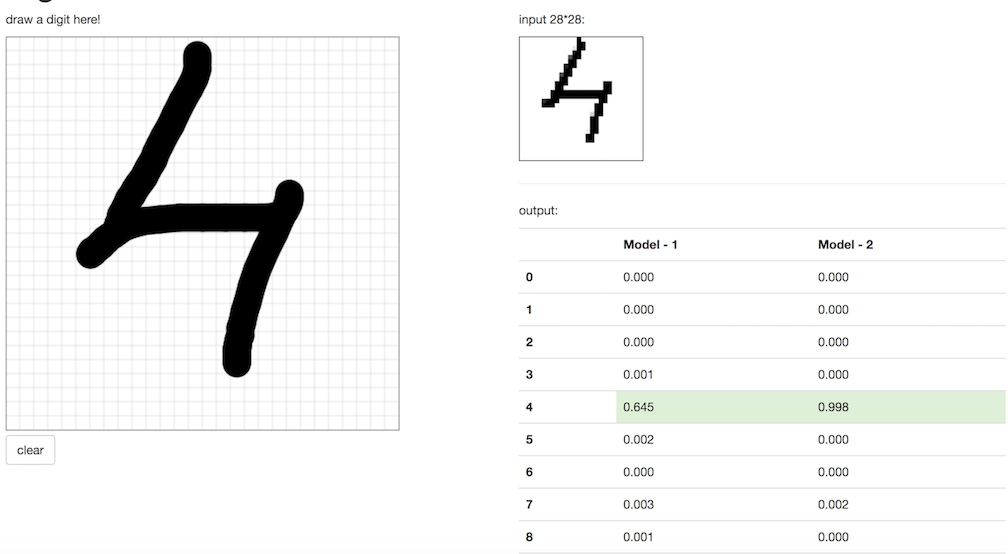Introduction
Architecture
Installation
Usage
Expansion
Videos

# Handwriting recognition with Tensorflow

## Introduction

The MNIST digit identification application provides an example of how to use source-to-image to build and deploy a tensorflow prediction endpoint on Openshift. Input data is taken from the MNIST site.

## Architecture

The system is composed of 3 pods:

• A front-end application: Which has a canvas where user can draw a digit and then the image is sent to 2 prediction endpoints.

• A Prediction Endpoint (regression): The tensorflow serving endpoint which serves a regression model.

• A Prediction Endpoint (cnn): The tensorflow serving endpoint which serves a CNN model.

Notebooks are available here regression and cnn.

## Installation

Installation of this application is using the `oc` command line tool. Once you are logged in to your OpenShift cluster, follow these instructions:

1. Ensure that you are connected to an OpenShift project

`oc new-project test`
2. Create the template for mnist web application.

`oc create -f https://raw.githubusercontent.com/sub-mod/mnist-app/master/template.json`
3. Create the template for tensorflow serving endpoints.

`oc create -f https://raw.githubusercontent.com/radanalyticsio/tensorflow-serving-s2i/master/template.json`
4. Launch tensorflow serving s2i build for the tensorflow models.We need to create two prediction endpoints.One for CNN and another for regression model.

```oc new-app --template=tensorflow-server --param=APPLICATION_NAME=tf-reg \
--param=SOURCE_REPOSITORY=https://github.com/sub-mod/mnist-models  \
--param=SOURCE_DIRECTORY=regression```
```oc new-app --template=tensorflow-server --param=APPLICATION_NAME=tf-cnn \
--param=SOURCE_REPOSITORY=https://github.com/sub-mod/mnist-models \
--param=SOURCE_DIRECTORY=cnn```
5. Launch the mnist web application with the two prediction endpoint service names.

```oc new-app --template=mnistapp --param=APPLICATION_NAME=mnist-app \
--param=PREDICTION_SERVICE1=tf-reg \
--param=PREDICTION_SERVICE2=tf-cnn```

## Usage

The `mnistapp` template creates a route on Openshift, so you should now be able to access the web application by clicking on the link provided in the UI. You can now begin drawing digits on the canvas and queries will be sent to the two prediction endpoints.The response from the prediction endpoints are displayed on the UI and should look similar to the following Image.